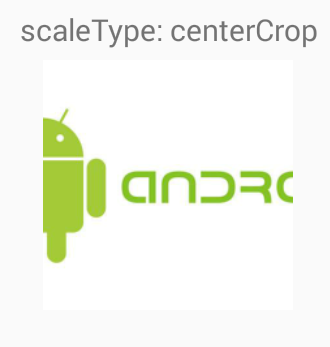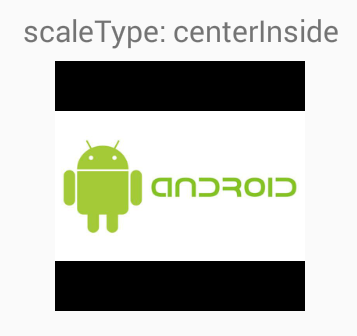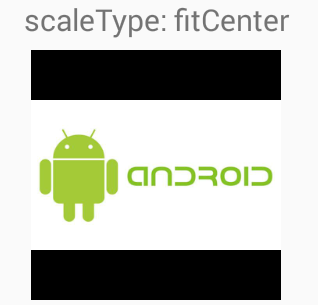# Set Scale Type

suggest change

Controls how the image should be resized or moved to match the size of `ImageView`.

XML attribute:

``android:scaleType="..."``

i will illustrate different scale types with a square `ImageView` which has a black background and we want to display a rectangular drawable in white background in `ImageView`.

``````<ImageView
android:id="@+id/imgExample"
android:layout_width="200dp"
android:layout_height="200dp"
android:background="#000"
android:src="@drawable/android2"
android:scaleType="..."/>``````

scaleType must be one of the following values:

1. `center`:Center the image in the view, but perform no scaling.1. `centerCrop`: Scale the image uniformly (maintain the image’s aspect ratio) so both dimensions (width and height) of the image will be equal to or larger than the corresponding dimension of the view (minus padding). The image is then centered in the view.1. `centerInside`: Scale the image uniformly (maintain the image’s aspect ratio) so that both dimensions (width and height) of the image will be equal to or less than the corresponding dimension of the view (minus padding). The image is then centered in the view.1. `matrix` : Scale using the image matrix when drawing.1. `fitXY`: Scale the image using FILL.1. `fitStart`: Scale the image using START.1. `fitCenter`: Scale the image using CENTER.1. `fitEnd`: Scale the image using END.